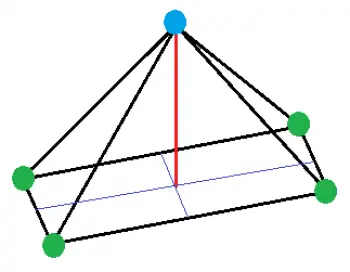# Types of Pyramids in Geometry: Classification CriteriaPyramids are three-dimensional geometric bodies that have a polygonal base and triangular faces that meet at a point, called a vertex. The properties of pyramids are applied in mathematics, architecture, engineering, and other fields.

The main elements of these geometric bodies are:

• Height: is the perpendicular distance from the vertex to the base.

• Base: is the flat geometric figure that serves as a support for the pyramidal structure and has a polygon shape.

• Lateral Faces: The lateral areas of a pyramid are the surfaces that extend from the sides of the base of the pyramid to the vertex of the pyramid. A pyramid has at least three lateral faces, but can have many more. All the lateral faces are isosceles triangles.

• Base Apothem: The apothem of a pyramid is the distance from the center of the base of the pyramid to the center of one of the lateral faces.

Pyramids can be classified in different ways according to the following criteria:

## Types of Pyramids According to the Shape of the Base

The most common types of pyramids according to their base are:

1. Triangular Pyramid: Triangular pyramids have a triangle-shaped base and side faces that are triangles.

2. Square pyramid: It has a square-shaped base.

3. Pentagonal pyramid: its base is shaped like a pentagon.

4. Hexagonal pyramid: its base forms a flat geometric figure in the shape of a hexagon.

5. Rectangular base pyramid.

## Types of Pyramids According to Regularity

Depending on the regularity of the polygons that form it, they can be classified as:

• Regular: A regular pyramid is one whose base is a regular polygon (polygon whose sides and angles are equal) and the lateral faces are congruent isosceles triangles. Therefore, both the lateral edges are equal.

• Irregular: An irregular pyramid is one whose base is an irregular polygon or which has lateral faces of different sizes and shapes.

## Types of Pyramids According to the Position of the Vertex

Depending on the position of the vertex with respect to its base, they can be classified as:

• Straight: A straight pyramid is one whose vertex is on the center of the base.

• Inclined: An oblique pyramid is one whose vertex is outside the center of the base.

## Properties of the Pyramids

Here are some of the most important properties of pyramids:

1. Height: The height of a pyramid is the perpendicular distance from the vertex to the base. The height determines the size of the pyramid and is used to calculate the area of ​​the base and the volume of the figure.

2. Volume: The volume of a pyramid is calculated using the formula V = (1/3)b·h, where “b” is the area of ​​the base and “h” is the height of the pyramid.

3. Area of ​​a Regular Pyramid: The total surface area of ​​a pyramid is the sum of the areas of all its faces. in the case of a regular pyramid, it is calculated using the formula S = b + (1/2) p h, where “b” is the area of ​​the base formed by a regular polygon and “p” is the perimeter of the base and “h” the height.

4. Relative height: The height of a pyramid with a regular base is always perpendicular to the plane of the base, and passes through the midpoint of the line connecting the vertex to the center of the base.

5. Vertex Angles: The sum of the angles at any vertex of a pyramid is always equal to 360 degrees.

6. Relation to prisms: A prism and a pyramid with the same base and height will have the same volume. Also, any pyramid can be split into a prism and a smaller pyramid, where the smaller pyramid has the same base and height as the original.

Author:

Published: April 18, 2023
Last review: April 18, 2023